# Kraken Daily Market Report for June 11 2020

## Overview

• Total trading volume ($302 million) much higher than the weekly average ($159 million) and the 30-day average ($190 million) – based on the 6% drop in Bitcoin over USD and EUR. Similar levels of loss were also seen in global equity markets. • Except for very small positive returns for some of the stable coins, most cryptos took 4% to 12% losses. • The best relative performer was Melon (MLN) -2.5% over USD, but up 3.5% over Bitcoin (XBT). June 11, 2020$301.5M traded across all markets today
Crypto, EUR, USD, JPY, CAD, GBP, CHF
XBT
$9290.3 ↓6.2%$216.7M
ETH
$230.74 ↓7.1%$39.1M
USDT
$0.9986 ↓0.18%$13.2M
$0.0751 ↓10%$5.95M
XTZ
$2.6145 ↓9.5%$5.56M
XRP
$0.1884 ↓7.1%$5.44M
BCH
$235.05 ↓8.6%$3.75M
$3.9690 ↓10%$2.75M
LTC
$43.289 ↓7.3%$1.89M
XLM
$0.0712 ↓9.6%$1.82M
USDC
$0.9999 ↑0.01%$1.82M
XMR
$63.694 ↓8.9%$1.61M
EOS
$2.5155 ↓8.9%$1.16M
REP
$13.829 ↓12%$825K
NANO
$1.0551 ↓11%$717K
DAI
$1.0056 ↑0.07%$707K
SC
$0.0026 ↓11%$654K
ATOM
$2.7666 ↓11%$635K
BAT
$0.2224 ↓11%$609K
ETC
$6.2625 ↓8.1%$499K
MLN
$4.6315 ↓2.5%$479K
ZEC
$49.810 ↓9.9%$479K
DASH
$72.289 ↓7.7%$473K
PAXG
$1726.2 ↓0.4%$334K
ALGO
$0.2308 ↓6.7%$310K
TRX
$0.0157 ↓11%$300K
ICX
$0.3202 ↓12%$298K
WAVES
$1.1343 ↓8.9%$281K
OMG
$1.5085 ↓8.5%$238K
QTUM
$1.6908 ↓9.4%$234K
OXT
$0.1803 ↓7.0%$201K
LSK
$1.1790 ↓9.4%$119K
GNO
$29.725 ↓5.3%$78.2K
XDG
$0.0024 ↓5.3%$55.3K

#####################. Trading Volume by Asset. ##########################################

The figures below break down the trading volume of the largest, mid-size, and smallest assets. Cryptos are in purple, fiats are in blue. For each asset, the chart contains the daily trading volume in USD, and the percentage of the total trading volume. The percentages for fiats and cryptos are treated separately, so that they both add up to 100%.

Figure 1: Largest trading assets: trading volume (measured in USD) and its percentage of the total trading volume (June 11 2020)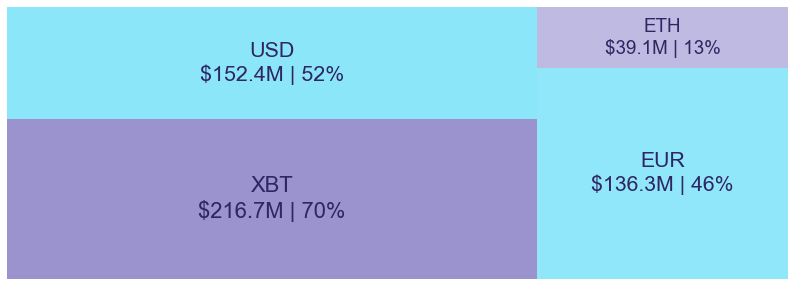Figure 2: Mid-size trading assets: (measured in USD) (June 11 2020)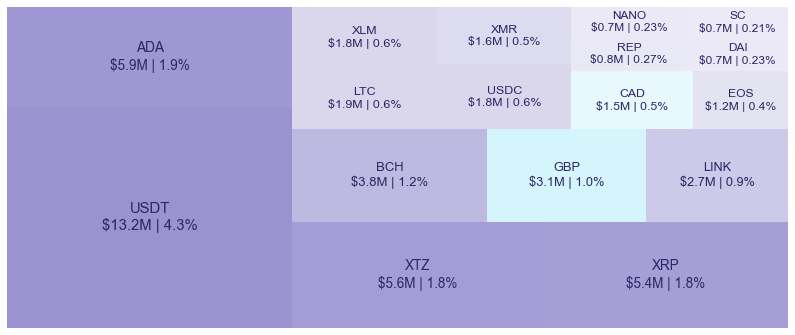Figure 3: Smallest trading assets: (measured in USD) (June 11 2020)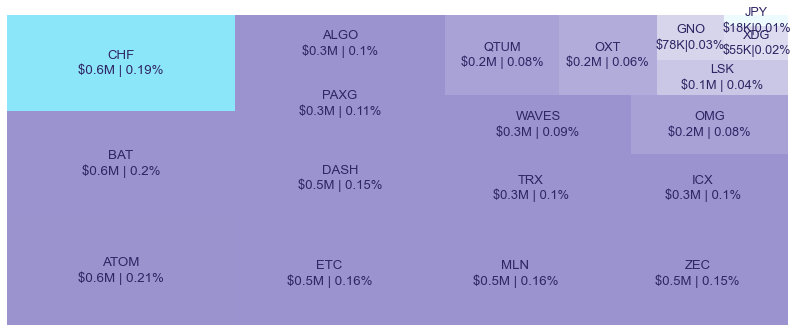Spread percentage is the width of the bid/ask spread divided by the bid/ask midpoint. The values are generated by taking the median spread percentage over each minute, then the average of the medians over the day.

Figure 4: Average spread % by pair (June 11 2020)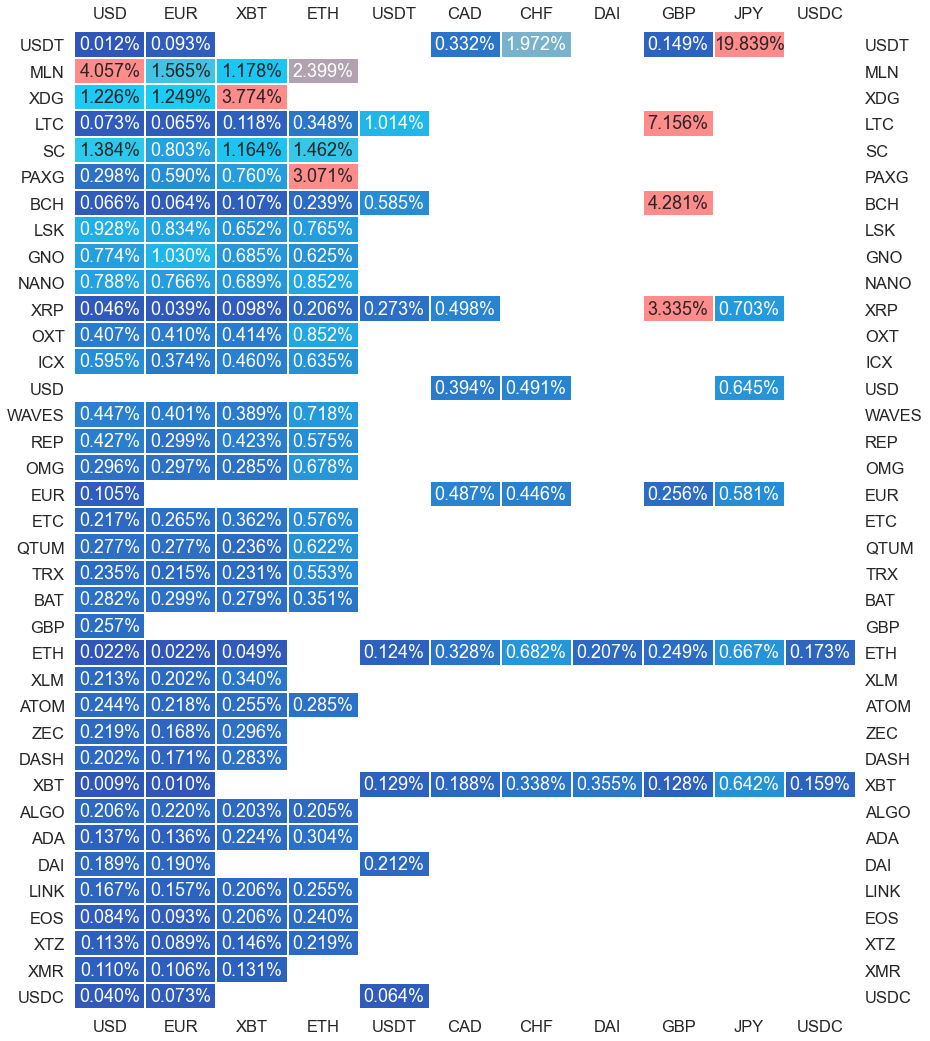.

#########. Returns and Volume ############################################

## Returns and Volume

Figure 5: Returns of the four highest volume pairs (June 11 2020)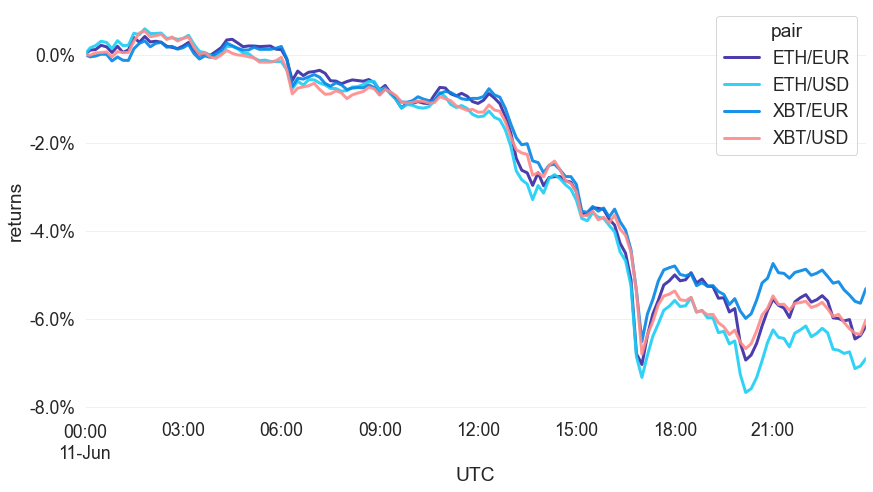Figure 6: Volume of the major currencies and an average line that fits the data to a sinusoidal curve to show the daily volume highs and lows (June 11 2020)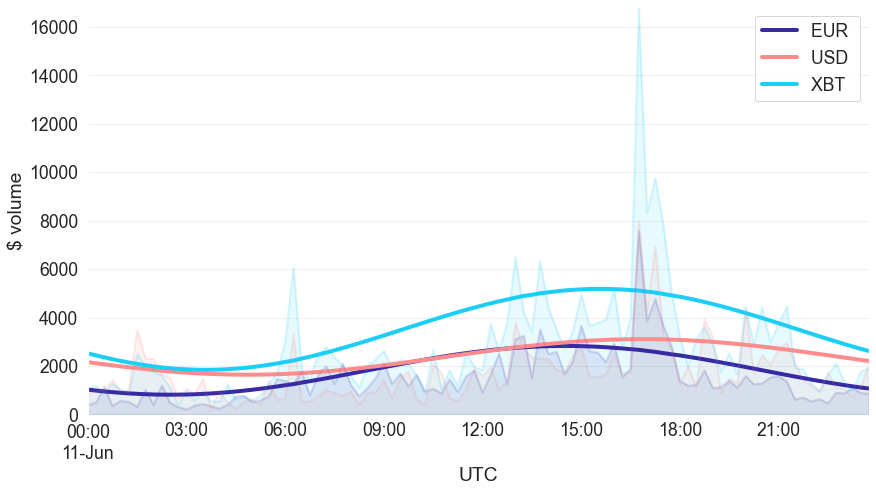###########. Daily Returns. #################################################

## Daily Returns %

Figure 7: Returns over USD and XBT. Relative volume is indicated by the size of the font. (June 11 2020)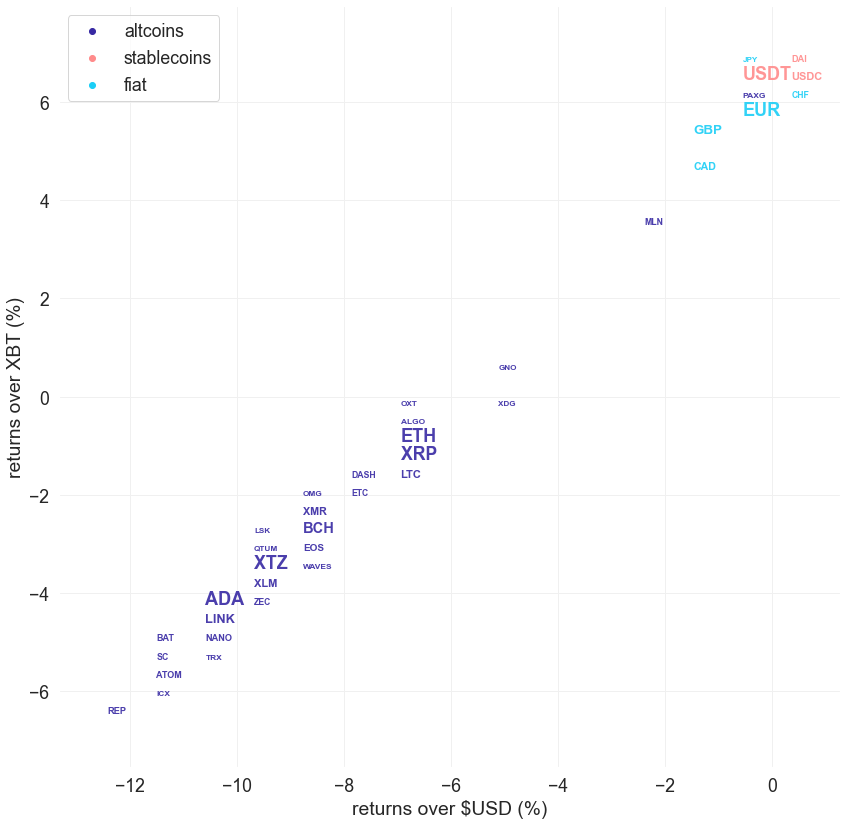###########. Disclaimer #################################################

The values generated in this report are from public market data distributed from Kraken WebSockets api. The total volumes and returns are calculated over the reporting day using UTC time.